Superconductivity

This free course is available to start right now. Review the full course description and key learning outcomes and create an account and enrol if you want a free statement of participation.

Free course

3.3 The London equations

A simple but useful description of the electrodynamics of superconductivity was put forward by the brothers Fritz and Heinz London in 1935, shortly after the discovery that magnetic fields are expelled from superconductors. Their proposed equations are consistent with the Meissner effect and can be used with Maxwell's equations to predict how the magnetic field and surface current vary with distance from the surface of a superconductor.

In order to account for the Meissner effect, the London brothers proposed that in a superconductor, Equation 4 is replaced by the more restrictive relationship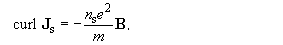This equation, and Equation 3 which relates the rate of change of current to the electric field, are now known as the London equations.

London equations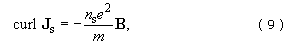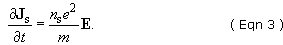It is important to note that these equations are not an explanation of superconductivity. They were introduced as a restriction on Maxwell's equations so that the behaviour of superconductors deduced from the equations was consistent with experimental observations, and in particular with the Meissner effect. Their status is somewhat similar to Ohm's law, which is a useful description of the behaviour of many normal metals, but which does not provide any explanation for the conduction process at the microscopic level.

To demonstrate how the London equations lead to the Meissner effect, we proceed in the same way as for the perfect conductor. First we use Ampère's law, curl B = μ﻿0﻿J﻿s, to substitute for J﻿s in Equation 9, and we obtain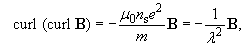where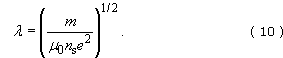But curl﻿(curl B﻿) = grad﻿(﻿div B﻿) − ∇﻿2﻿B = −﻿∇﻿2﻿B, since div B = 0. So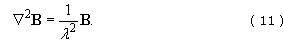This equation is similar to Equation 7, but ∂﻿B/∂﻿t has been replaced by B. The important point to note about this equation is that the only solution that corresponds to a spatially uniform field (for which ∇﻿2﻿B = 0﻿) is the field that is identically zero everywhere. If B were not equal to zero, then ∇﻿2﻿B would not be zero, so B would depend on position. Thus, a uniform magnetic field like that shown in Figure 10b cannot exist in a superconductor.

If we consider again the simple one-dimensional geometry shown in Figure 14, then we obtain the solution to Equation 11 by simply replacing the partial time derivatives of the fields in the solution for the perfect conductor (Equation 8) by the fields themselves, that is,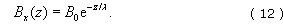Therefore, the London equations lead to the prediction of an exponential decay of the magnetic field within the superconductor, as shown in Figure 15.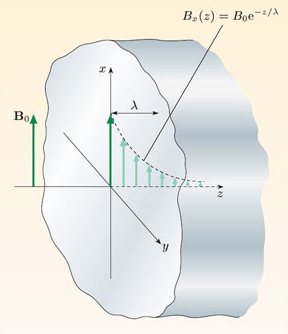Figure 15 The penetration of a magnetic field into a superconducting material, showing the penetration depth, λ.

SMT359_1